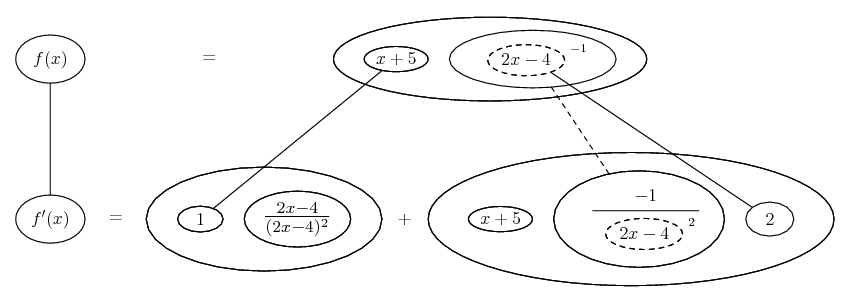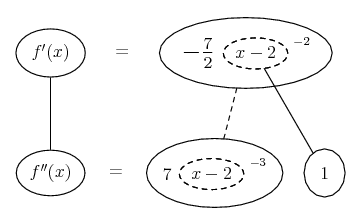# Thread: Derivatives of Rational Functions

1. ## Derivatives of Rational Functions

Find the First and Second Derivative of:

f(x)=(x+5)/(2x-4)

Rewrite and use the product rule:

f(x)=(x+5)(2x-4)^-1
f'(x)=(x+5)((2x-4)^-1)' + (x+5)'(2x-4)^-1
f'(x)=(-2)(2)(x+5)(2x-4)^-2+(1)(2x-4)^-1

After Simplifying:

f'(x)=-4x^2-12x+40/(2x-4)^2

At this point do I simplify further by making the denominator a polynomial or do I keep it within it's brackets?

f'(x)=-4x^2-12x+40/(2x-4)(2x-4)
f'(x)=-4x^2-12x+40/4x^2+16

Then do I divide the numerator and denominator?

f'(x)= x^2-12x+(5/2)

I have to find the second derivative, so I want to simplify as far as possible to make finding the second derivative easier.

thanks2.Originally Posted by ButumFind the First and Second Derivative of:

f(x)=(x+5)/(2x-4)

Rewrite and use the product rule:

f(x)=(x+5)(2x-4)^-1
f'(x)=(x+5)((2x-4)^-1)' + (x+5)'(2x-4)^-1
f'(x)=(-2)(2)(x+5)(2x-4)^-2+(1)(2x-4)^-1 <<<<<<<<< the factor (2) is wrong

After Simplifying:

f'(x)=-4x^2-12x+40/(2x-4)^2

At this point do I simplify further by making the denominator a polynomial or do I keep it within it's brackets?

f'(x)=-4x^2-12x+40/(2x-4)(2x-4)
f'(x)=-4x^2-12x+40/4x^2+16

Then do I divide the numerator and denominator?

f'(x)= x^2-12x+(5/2)

I have to find the second derivative, so I want to simplify as far as possible to make finding the second derivative easier.

thanksIf you re-write the equation of the 1st differentiation you'll get:

\displaystyle \begin{aligned} f'(x)&= (-2)(x+5)(2x-4)^{-2}+(1)(2x-4)^{-1}\\ &=\frac{-2(x+5)}{(2x-4)^2}+\frac1{2x-4}\\ &=\frac{-2(x+5)}{(2x-4)^2}+\frac{2x-4}{(2x-4)^2}\\ &=- \frac7{2(x-2)^2}\end{aligned}

And now the 2nd differentiation is quite easy.

3. Whoops I actually meant:

f(x)=(x+5)(2x-4)^-1
f'(x)=(x+5)((2x-4)^-1)' + (x+5)'(2x-4)^-1
f'(x)=(-1)(2)(x+5)(2x-4)^-2+(1)(2x-4)^-1

I'm still shaky on the chain rule. But do I not need to find the derivative for (2x-4) = 2?

4. Just in case a picture helps...... where... is the chain rule, here wrapped inside the legs-uncrossed version of...... the product rule. Straight continuous lines differentiate downwards (integrate up) with respect to x, and the straight dashed line similarly but with respect to the dashed balloon expression (the inner function of the composite which is subject to the chain rule).

Spoiler:_________________________________________

Don't integrate - balloontegrate!

Balloon Calculus: Standard Integrals, Derivatives and Methods

Balloon Calculus Drawing with LaTeX and Asymptote!

5. Thank you for the balloons Tom, it helped.

derivatives, functions, rational